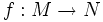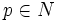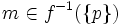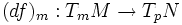# Regular value

Let$f:M \to N$ be a smooth map of differential manifolds. A point$p \in N$ is termed a regular value of$f$ if for every$m \in f^{-1}(\{p\})$, the induced map$(df)_m: T_mM \to T_pN$, is surjective.